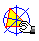TRIPOLAR CENTROID

Let P be a point, L its tripolar. Let P' be the infinite point of  L, and L' the tripolar of P'. The intersection between L and L' is the point TG(P) called the tripolar centroid of P.Tripolar_centroid.macTripolar_infinite_centroid.mac